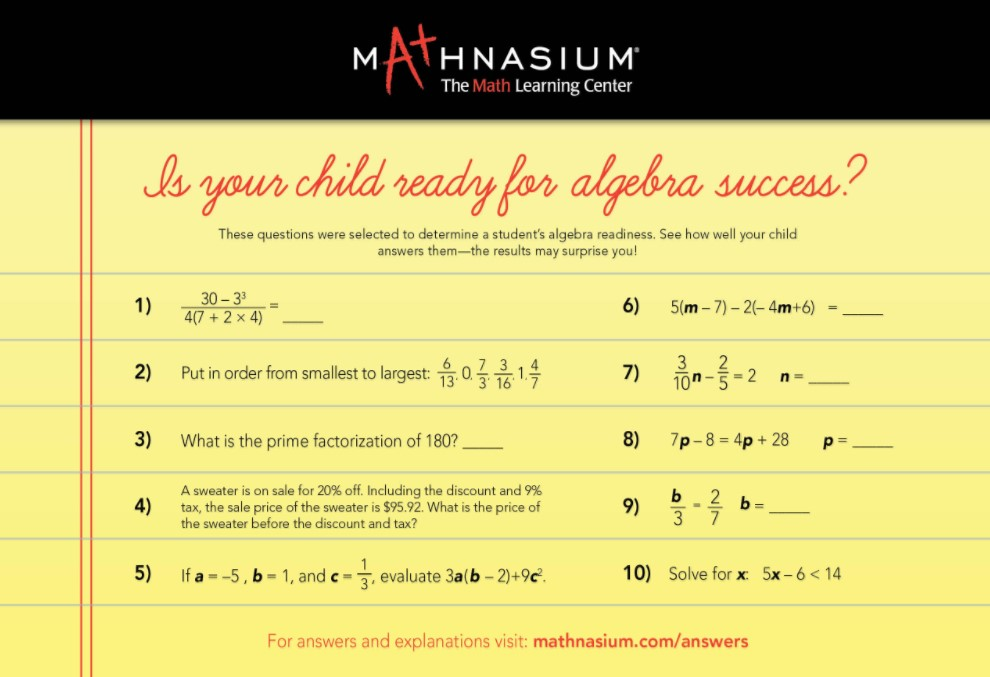* indicates a required field• phone number format invalid• email format invalidProblems detected, please review the form.
Privacy - Terms

# News from Mathnasium of Nolensville

### Mathnasium of Nolensville - Algebra Tutor and Algebra Homework Help

Oct 27, 2020

In middle school and high school, students focus on higher math, including Algebra I and Algebra II.  Students in Williamson County Schools taking these subjects will be working towards Advanced Placement (AP) exams, Tennessee Comprehensive Assessment Program (TCAP), End of Course (EOC) exams, and standardized college entrance exams such as the SAT and the ACT.

Related Topics:

When students reach Algebra, it is a culmination of the fundamental skills from all math classes in elementary and middle school; and sets the stage for all high school and college math classes that will follow.

Often, students come to Mathnasium of Nolensville in need of Algebra tutoring and instruction because of gaps in the prerequisite math curriculum necessary to make sense of the material covered in Algebra class.

Getting ready for Algebra starts in first grade, with each year of math building on the prior through elementary school and beyond.  Algebra presents students with the more abstract side of math. In order to make sense of these abstractions, they need to master their number facts and fractions, build their computational skills, and gain number sense (an intuitive understanding of how numbers work). This goes beyond memorizing algorithmic processes.

Our Approach

To determine each student’s math abilities, Mathnasium of Nolensville gives a written and verbal assessment exclusive to the Mathnasium Method. Based on the diagnostic assessment results, our math instructors build customized learning plans designed to fit the student’s specific needs in two important ways -- avoid spending time on things they already know and strengthen the things they need help with to reduce math frustration.

We understand the pressures and busy schedules our Nolensville and Williamson County students face.  Our focus is to help with immediate problems, such as homework help and test prep, while filling in any foundational gaps in their mathematical knowledge. The goal is to enhance their performance in the crucial exams they’ll be taking that can have a great effect on their future: Tennessee Comprehensive Assessment Program (TCAP), End of Course (EOC) exams, Advanced Placement (AP) exams, and the SAT and the ACT.

Algebra Homework Help

Our experienced Algebra tutors and instructors provide algebra tutoring, both classwork and homework help, that addresses gaps in knowledge. As these gaps close, students understand the material better, boost homework confidence, and raise their Algebra class grades. With this help, instead of seeing Algebra homework as a burden and frustration, students begin to see it as a welcome challenge and an opportunity for further learning.

Mathnasium of Nolensville Algebra Tutor and Instruction

The curriculum samples shown here represent critical topics we address:

Algebra 1

• Connecting algebra to previous learning

• Solving linear equations, systems of linear equations (2 variables), and quadratic equations

• 2(5x - 1) = 3x + 3

• 4y + 3 = 0

• x^2 - x - 1 = 0

• Factoring:

• x^2 - 8x - 2

• a^2 - 36

• 9x^2 - x - 8

• 12abc - 8ab - 4a

• Solving algebraic word problems

• "30 stamps are worth a total of \$8.61. Some of the stamps are worth 32¢ each and the rest are worth 23¢ each. How many of the stamps are worth 23¢ each?"

Algebra 2

• The relatioship between logs and exponents

• Arithmetic and geometric sequenes

• "What is the 10th term of the sequence: 1, 1/2, 1/4, 1/8, ... ?"

• Combinations and permutations

• "How many ways can 7 books be arranged on a shelf in groups of 5?"

• Matrix operations

• Study of complex numbers

• Simplify: (2 + 3i)/(3 - 4i)

Preparing for Algebra

Here are some of the math concepts and skills students need to master in preparation for Algebra 1:

• Fluency with basic math operations (addition, subtraction, multiplication, and division)
• A solid understanding of fractions, percents, and decimals -- and how they're all related
• Ratio and proportion
• Probability
• Geometry formulas and vocabulary: perimeter, area, circumference, etc.
• Integers, the number line, and integer operations

... as you can see, many of these relate to topics covered in the early elementary grades!

If you’re curious about how prepared your student is for Algebra, review these questions with them: